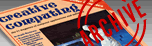`CREATIVE COMPUTING VOL. 9, NO. 5 / MAY 1983 / PAGE 196`

 The apocalypse equations. Howard S. Balsam. The Apocalypse Equations The last book of the Bible is that of Revelation or Apocalypse. It describes the end of this world with the Battle of Armageddon and the advent of the next and perfect world. Engendered by the growth of nuclear weaponry over the last 35 years and by massive and skillful Kremlin-fanned propaganda, there is a widespread fear of starting Armageddon by accidentally launching an armed nuclear missile from either the United States or Soviet Russia. In the Harvard Magazine for March/April, 1982 appeared a letter by one Bradford Lyttle, of Chicago, presenting three forms of an equation which he says gives the probability of such an accidental Armageddon. The Apocalypse program, which follows, presents these equations so you may investigate the presumed probability of Apocalypse. Probability is often expressed as a decimal between zero and one. A probability near zero indicates that the changes of a given event occurring are considered very low. A probability near one says that the chances are very high that the event will occur. Probabilities exceeding about 0.95 are often considered practically certain. Probability is expressed as a percentage in this program: 100%=1.00, a certainty. The Equations The three equations use these symbols: AP is the Probability of Apocalypse, that is, the probability over a period of time that the accidental launch of one nuclear missile by either the United States or the Soviet Union would falsely trigger a nuclear World War III. U is the total number of strategic missiles in the U.S. arsenal. The program suggests 1900. You may wish to adjust this. S is the total number of strategic missiles in the Soviet arsenal. The program suggests 2200; again, you may adjust this value. P is the probability of the accidental launching of an armed strategic nuclear missile by either the U.S. or the Soviet Union during any 24-hour period. The program suggests that there is one chance in one hundred million of this: 1E-8. Change this if desired. N is the number of days in the period considered. You may enter whatever period you wish: the program suggests 14,600 days--40 years. You may consider that the clock started ticking perhaps 20 years ago, with a much smaller number of missiles. The three equations given by Mr. Lyttle are: Approximate: AP = 1-(1-P(U + S))N Closer approximation: AP=1-e(-NP(U+S)) Exact Form: AP=1-(1-P)(N(U+S)) It turns out that the values given by the first two equations are usually very close to one another, while the exact equation gives a slightly smaller value. Program Structure The Applesoft program consists of 14 sections or routines ranging from 1 to 12 lines each in length. In approximate order of operation, the main routines are: 1000-1090 Introduction 800- 890 Menu 600- 720 Entries 300- 370 Approximate Equation 400- 470 Closer Approximation 500- 570 Exact Equation 140- 180 Results Brief routines handle such operations as centering phrases on the screen, rounding numbers, rejecting out-of-line values, and beeping three times. The Entries subroutine is designed to minimize number re-entries; after the initial entries, each re-entry with the same value can be made with one keystroke. The program is fairly well bullet-proofed and includes many REMarks. Variations While the program considers strategic missiles of the U.S. and Soviet Russia only, the nuclear "club' is growing. You may modify the program accordingly. You may wish to take a short-cut, at least for a minimum-effort trial. If so, try skipping lines 1000-1090 (Introduction). If you do this, make line 10 read: GOTO 810. Of course, you may also omit the REMs. If you find the beeps annoying, delete lines 70-80 and GOSUB 80 in lines 200, 350, 450, 550, 890 and 1100. Now, RUN the program and find out what the future holds--if anything. Table: